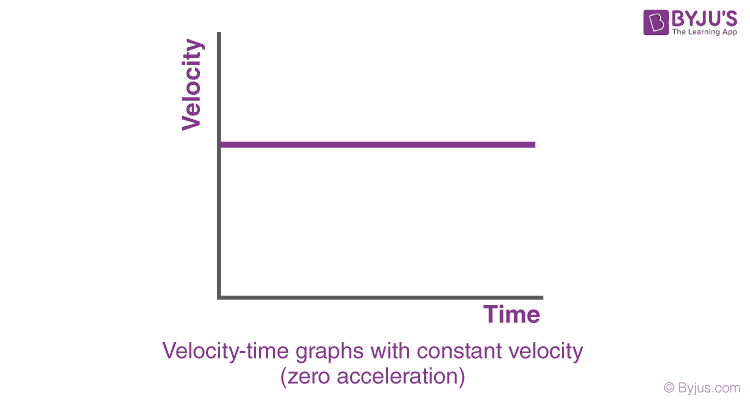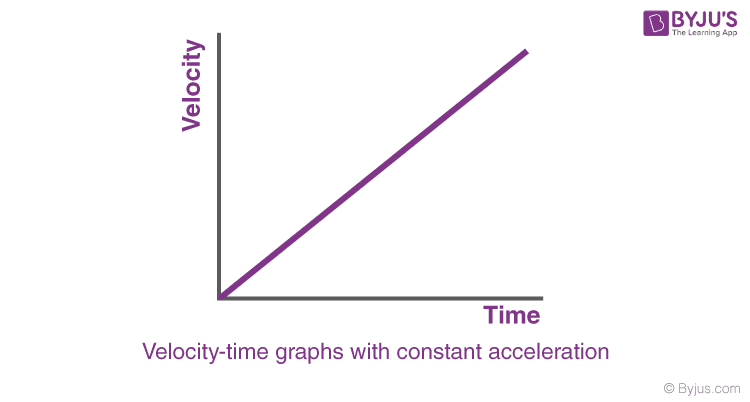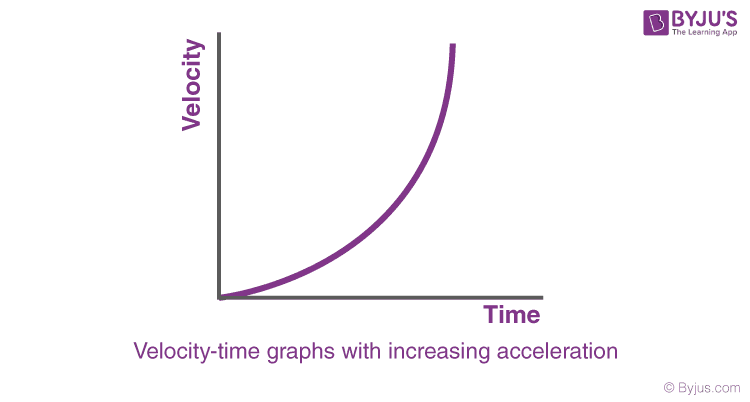Sign-up for an Intro to Teaching with 3D and VR workshop here

RegisterSwati Talwar

HS-PS2-1

# Velocity vs. Time Graph Study Guide

A velocity vs. time graph gives a lucid representation of the speed and direction of an object in a time period.

# INTRODUCTION

Acceleration is the sensation you have when you're sitting in a jet during take-off, hitting on the brakes in a car, or turning a sector at a great velocity in a go-kart. This feeling of acceleration is felt due to a change in the velocity of these motions.

## VELOCITY vs. TIME GRAPH

• The meaning of velocity is an object's speed in a specific direction.

• The velocities of two vehicles going at the same speed but also in different directions are distinct.

• A velocity vs. time graph represents the speed and direction of an object over a certain time period.

• Speed-time graphs are sometimes known as velocity vs. time graphs.

• The velocity of the item is the vertical axis of a velocity vs. time graph.

• The time period from the beginning of the motion is represented on the horizontal axis.

## SLOPE AND THE AREA IN A VELOCITY vs. TIME GRAPH

• The object's acceleration is represented by the gradient/slope of a velocity vs. time graph.
• As a result, the value of the slope at a given moment shows the object's acceleration at that time.
• This implies that whenever the gradient is steep, the object's velocity will change quickly and in cases where the slope is low, the object's velocity does not change as quickly.
• This also indicates that the acceleration will be negative if the slope is negative (oriented downwards) and positive if the gradient is positive (oriented upwards).
• Also, the region under the graph represents an object’s displacement in a velocity vs. time graph.

## FEATURES OF VELOCITY vs. TIME GRAPH

Velocity vs. time graph with zero acceleration

In this case, the velocity would be constant throughout the time interval of the motion, no matter how much the time changes.Source

Velocity vs. time graph with constant acceleration

Whenever the acceleration is constant (positive), and the initial velocity of an object is zero, the object's velocity increases linearly.Source

Velocity vs. time graph with increasing acceleration

The velocity vs. time graph will be a curve when the acceleration increases with time.Source

# SUMMARY

• A velocity-time graph depicts how the velocity of an object in motion changes over time.
• The gradient/slope of a velocity vs. time graph shows the moving object's acceleration.
• The region under the graph represents an object’s displacement in a velocity vs. time graph.

## FAQs

1. How to make a velocity vs. time graph?

We can make a velocity vs. time graph by plotting the velocity on the y axis and the time on the x-axis.

2. How to draw a velocity vs. time graph?

By plotting the velocity of a motion on the vertical axis and at the time from the beginning on the horizontal, we can draw a velocity vs. time graph.

3. What is the distance between two points using the velocity vs. time graph?

The area under the graph of the two points on the velocity vs. time graph depicts the displacement between them.

We hope you enjoyed studying this lesson and learned something cool about Velocity vs. Time Graph! Join our Discord community to get any questions you may have answered and to engage with other students just like you! We promise, it makes studying much more fun!😎

## REFERENCE

1. Velocity vs. Time Graphs: https://flexbooks.ck12.org/cbook/ck-12-physics-flexbook-2.0/section/2.9/primary/lesson/velocity-time-graphs-ms-ps/. Accessed 8th April 2022.
2. Velocity vs. Time Graphs: https://www.khanacademy.org/science/physics/one-dimensional-motion/acceleration-tutorial/a/what-are-velocity-vs.-time-graphs Accessed 8th April 2022.